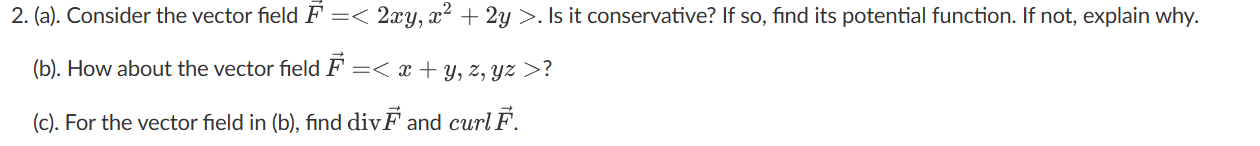Home / Expert Answers / Physics / 2-a-consider-the-vector-field-f-lt-2xy-x2-2y-gt-is-it-conservative-if-so-find-its-potentia-pa735

# (Solved): 2. (a). Consider the vector field F=<2xy,x2+2y>. Is it conservative? If so, find its potentia ...2. (a). Consider the vector field . Is it conservative? If so, find its potential function. If not, explain why. (b). How about the vector field ? (c). For the vector field in (b), find and curl .

We have an Answer from Expert